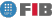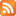# Operations Research Models for Decision Making (MIOPD)

Credits Dept. Type Requirements
7.5 (6.0 ECTS) EIO
• Elective for DIE
• Elective for DCSFW
AL - Prerequisite for DIE , DCSYS
PRED - Prerequisite for DIE , DCSYS

## Instructors

 Person in charge: (-) Others: (-)

## General goals

This subject presents some of the fundamental methods of operations research. Operations research is a discipline based on the formulation of models and the development and application of algorithms for solving problems in decision making. The subject also goes into the modelling languages and software vital to effective problem solving. The subject has a practical orientation. Students are introduced to several applications of the model and algorithms that may come into play complex problems they will come across in the future, both in technical and managerial fields.

## Specific goals

### Knowledges

1. Model concept. Linear models, network flows, integers and non-linear numbers.
2. Simplex algorithm for linear programming.
3. Simplex algorithm for the minimum cost problem in network flows.
4. Shortest path algorithms for positive costs (Dijkstra) and label-correcting.
5. Algorithms for the maximum flow problem.
6. The branch-and-bound algorithm for integer programming.
7. Basic properties of non-linear problems.
8. Heuristic methods for solving sequencing problems, sequencing, itneraries, etc.
9. Dynamic programming.
10. Learn how to formulate and solve problems using modelling and optimisation software.

### Abilities

1. Learn how to identify Mathematical Programming/Optimisation problems.
2. Ability, given a problem, to model it and determine whether it is linear, non-linear or entire.
3. Solve a model using appropriate software modelling and algorithms. Interpret the solution.
4. Learn how to use software modelling and optimisation packages.

### Competences

1. Solve decision-making problems with the aid of computers.
2. Ability to summarise, logical reasoning, and solution of problems using quantitative techniques.
3. Formulate and solve (albeit approximately) difficult computational problems (NP-complets).
4. Use biography and software written in English.

## Contents

Estimated time (hours):

 T P L Alt Ext. L Stu A. time Theory Problems Laboratory Other activities External Laboratory Study Additional time

1. Introduction.
T      P      L      Alt    Ext. L Stu    A. time Total
1,0 0 0 0 0 0 0 1,0
- Operational research, Mathematical Programming and Optimisation. Models.

- Classification of problems and solution algorithms.

2. Modelling and modelling languages.
T      P      L      Alt    Ext. L Stu    A. time Total
8,0 4,0 10,0 0 10,0 10,0 0 42,0

- Linear models, nonlinear integer models. Examples and case studies.

- The AMPL modelling language.

The lab sessions will demonstrate the Excel and AMPL modelling environments.

Five case studies are presented in the theory and lab sessions:

- Management of time-limited projects (linear programming).

- Maximum network flows.

- Itinerary problems: TSP (full programme).

- design de networks (full programme).

- Optimisation of a Support Vector Machine (non-linear programming, quadratics).

These two cases will be be used in calculating the grade for the practical work.

3. Linear programming.
T      P      L      Alt    Ext. L Stu    A. time Total
8,0 2,0 2,0 0 0 8,0 0 20,0
Basic properties of linear programming.

- Computational complexity of linear programming.

- The Simplex algorithm (first version, matricial format).

4. Linear problems exhibiting a network structure: network flows.
T      P      L      Alt    Ext. L Stu    A. time Total
10,0 4,0 4,0 0 0 12,0 0 30,0

- The theory sessions will cover:

- Basic properties of problems exhibiting a network structure:

- Types of network flow models. Examples.

- Simplex algorithm for the minimum cost problem in network flows.

- Shortest path algorithms for positive costs (Dijkstra) and label-correcting").

Algorithms for the maximum flow problem.

5. Integer programming.
T      P      L      Alt    Ext. L Stu    A. time Total
4,0 1,0 1,0 0 0 4,0 0 10,0
Branch and bound algorithm.

6. Non-linear programming
T      P      L      Alt    Ext. L Stu    A. time Total
4,0 2,0 0 0 0 4,0 0 10,0
Basic properties of non-linear programming.

7. Heuristic methods
T      P      L      Alt    Ext. L Stu    A. time Total
6,0 2,0 0 0 0 6,0 0 14,0

- Heuristic methods for solving the sequencing of tasks.

- Heuristic methods for designing networks.

- Heuristic methods for tackling itineraries.

8. Dynamic programming.
T      P      L      Alt    Ext. L Stu    A. time Total
2,0 0 0 0 0 1,0 0 3,0

 Total per kind T P L Alt Ext. L Stu A. time Total 43,0 15,0 17,0 0 10,0 45,0 0 130,0 Avaluation additional hours 5,0 Total work hours for student 135,0

## Docent Methodolgy

This will include theory, problem, and lab sessions. The basic knowledge contained in the course will be introduced in the theory sessions. The problems dealt with in the practical sessions will be directly based on the theory taught. The lab sessions will involve solving real-life medium-sized cases in which students will formulate and solve problems using modelling software and appropriate "solvers".

## Evaluation Methodgy

The final grade (NF) is calculate thus: NF= 0.7*NT+0.3*NP, with NT being the theory grade and NP the grade for the practical work.

NT: This part exam will be be calculated as NT/2 where students obtain a grade of 4 or over. The final exam will be calculated as NT/2 for those students who obtained a grade of 4 or higher, and as NT for the remaining students.

NP: There will be two practical assignments. These will be carried out during class time in the lab. Each assignment will consist of modelling and solving a case using AMPL modelling-optimisation software. Each practical assignment will be marked as NP/2.

## Basic Bibliography

• Ravindra K. Ahuja, Thomas L. Magnanti, James B. Orlin Network flows : theory, algorithms, and applications, Prentice Hall, 1993.
• Robert Fourer, David M. Gay, Brian W. Kernighan AMPL : a modeling language for mathematical programming, Thomson/Brooks/Cole, 2003.
• Jorge Nocedal, Stephen J. Wright Numerical optimization, Springer,, 1999.
• Wayne L. Winston Operations research : applications and algorithms, Brooks/Cole - Thomson Learning, 2004.
• L.A. Wolsey Integer programming, John Wiley & Sons, 1998.

## Complementary Bibliography

• Dimitris Bertsimas, John N. Tsitsiklis Introduction to linear optimization, Athena Scientific, 1997.
• Cliff T. Ragsdale Spreadsheet modeling and decision analysis : a practical introduction to management science, South-Western Publishing, 2001.

1. http://www-neos.mcs.anl.gov/

2. http://www.ece.northwestern.edu/OTC/

3. http://plato.la.asu.edu/guide.html

## Previous capacities

Students will need have acquired basic knowledge of the following from previous courses. In particular, basic knowledge of:

- programming
- algebra (operations with matrices and vectors)
- data structures for graphs
- Basic algorithms for graphs.© Barcelona school of informatics - Contact -RSS
This website uses cookies to offer you the best experience and service. If you continue browsing, it is understood that you accept our cookies policy.
Classic version Mobile version